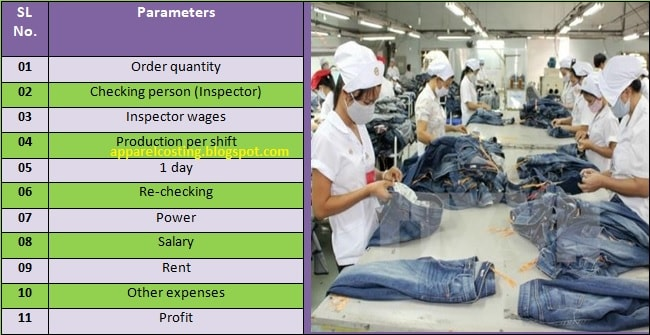# Apparel Costing

Solution of textile and apparel calculation

## How to Calculate Apparel Checking or Inspection Cost in Clothing Industry?

Estimation of Apparel Checking Cost:
The person who responsible for the apparel checking or inspection cost calculation has to know some key factors of apparel checking cost such as cost of re-checking, transportation, power charges etc. these will definitely help him in apparel checking cost calculation.Fig: Apparel inspection cost calculation process
Apparel Inspection Cost Calculation Method:
Calculate apparel checking or inspection cost and rate by following the given below chart.

 SL No. Parameters Quantity & Cost 01 Order quantity 10000 pcs apparel 02 Checking person (Inspector) 10 03 Inspector wages Rs. 125 per shift 04 Production per shift 2000 pcs apparel 05 1 day 2 shift (1 shift= 8hrs) 06 Re-checking 1000 pcs (Person- 02, Time- 03 shifts) 07 Power Rs. 5000 per month 08 Salary Rs. 20000 per month 09 Rent Rs. 10000 per month 10 Other expenses Rs. 15000 per month 11 Profit 10%

Solution:
The below has needed in apparel checking cost calculation:

Apparel checking cost per apparel,

Direct material cost + Direct labor cost + Direct expense cost + Overhead cost
= …………………………………………………………………….....…...........……......    ...… (1)
Total checking production

Total Checking Production Calculation:

Total production = 10000 pcs apparel

Total Checking Production Time Calculation:

Total production time,
= (Total checking production / Production per shift)
= (10000/2000) shift
= 5 shifts

Direct Material Cost Calculation:

Direct material cost = 0

Direct Labor Cost Calculation:
Operator wage,
= (Number of labor × Number of shifts × operator wage per shift)
= 10×5× Rs. 125
= Rs. 6250

Direct Expense Cost Calculation:

Re-checking labor,
= (No. of labors × No. of shifts × operator wage per shift)
= 2×3× Rs. 125
= Rs. 750

In power cost calculation,
= (Cost × 5 shifts) / (30days × 2 shift in a day) = {(50000 × 5)/60} = Rs. 417

In rent cost calculation,
= (Cost × 5 shifts) / (30days × 2 shift in a day) = {(10000 × 5)/60} = Rs. 834

In other expenses cost calculation,
= (Cost × 5 shifts) / (30days × 2 shift in a day) = {(15000 × 5)/60} = Rs. 1251

In salary cost calculation,
= (Cost × 5 shifts) / (30days × 2 shift in a day) = {(20000 × 5)/60} = Rs. 1667

So,

= Rs. 417 + Rs. 834 + Rs. 1251 + Rs. 1667
= Rs. 4172

Now,
From equation-01, we get,

Apparel checking cost per pc,

(Rs. 0 + Rs. 6250 + Rs. 750 + Rs. 4172)
= …………………………………………………..
10000

= Rs. 1.10

Apparel checking rate stands at
= {1.10/ (1-10%)}
= Rs. 1.25

You may follow:
1. How to Calculate Apparel Costing for Brief or Shorts?
2. How to Calculate Apparel Costing for T-Shirt?
3. 4 Steps of Apparel Costing in Clothing Manufacturing Factory
4. How to Calculate Apparel Costing for Boxer Shorts?
5. How Textile and Apparel Waste Produced in Manufacturing Factory?
6. List of Top 10 Textile Companies in World